# Andre

Andre, Thomas, and Ivan split 88 postage stamps in a 2:5:4 ratio. How much did Thomas get?

t =  40

### Step-by-step explanation: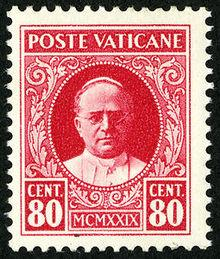Did you find an error or inaccuracy? Feel free to write us. Thank you!Tips to related online calculators
Need help to calculate sum, simplify or multiply fractions? Try our fraction calculator.
Check out our ratio calculator.

## Related math problems and questions:

• Money split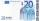Anton and Ferko got 2,500 euros together. The amount was split in a 1:4 ratio. How much did each get?
• Trio ratioHans, Alena and Thomas have a total of 740 USD. Hans and Alena split in the ratio 5: 6 and Alena and Thomas in the ratio 4: 5. How much will everyone get?
• Two brothersThe two brothers were to be divided according to the will of land at an area of 1ha 86a 30m2 in a ratio of 5:4. How many will everyone get?
• PointsGryffindor won 437 points. How many points obtained by each of the faculties if they were split at a ratio of 5: 7: 3: 4?
• Division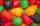Three siblings Helena, Oliver and George split the bag with candies on merit in the ratio 6:1:4. How many candies should each get if in bag were 88?
• Cyclist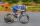A cyclist passes 88 km in 4 hours. How many kilometers did he pass in 8 hours?
• GardenThe rectangular garden has dimensions of 27 m and 30 m. Peter and Katka split it in a ratio of 4:5. How many square meters did Katkin measure part of the garden?
• Two gardenersThe garden with an area of 81 square meters was divided by two gardeners in a ratio of 4: 5. How much did the second gardener get more first?
• Divide moneyDivide 1200 USD at a ratio of 1:2:3:4:5:6:9:10
• Ratio - proportion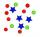Reduce the number 16 in proportion 3:2 5:4 11:8
• Fractions and mixed numerals(a) Convert the following mixed numbers to improper fractions. i. 3 5/8 ii. 7 7/6 (b) Convert the following improper fraction to mixed number. i. 13/4 ii. 78/5 (c) Simplify these fractions to their lowest terms. i. 36/42 ii. 27/45 2. evaluate following ex
• Divide 5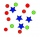Divide 288 in the following ratio 3 : 4 : 5
• Cupcakes 2Susi has 25 cupcakes. She gives 4/5. How much does she have left?
• RatiosDivide: a) 250 CZK in the ratio 2:3 b) 1000 CZK in the ratio 4:7:9
• Moneys in triple ratioMilan, John and Lili have a total 344 euros. Their amounts are in the ratio 1:2:5. Determine how much each of them has?
• Between two mixed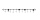What is the rational number between 2 1/4 and 2 4/5?
• Candies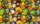There are 150 candies in the bowl. Ivan took 7 thirtieths, Charles 6 fifteenths, and Thomas 3 tenths. How many sweets are left in the bowl?